# Title 40

## SECTION 1066.605

### 1066.605 Mass-based and molar-based exhaust emission calculations.

§ 1066.605 Mass-based and molar-based exhaust emission calculations.

(a) Calculate your total mass of emissions over a test cycle as specified in paragraph (c) of this section or in 40 CFR part 1065, subpart G, as applicable.

(b) See the standard-setting part for composite emission calculations over multiple test intervals and the corresponding weighting factors.

(c) Perform the following sequence of preliminary calculations to correct recorded concentration measurements before calculating mass emissions in paragraphs (e) and (f) of this section:

(1) For vehicles above 14,000 pounds GVWR, correct all THC and CH4 concentrations for initial contamination as described in 40 CFR 1065.660(a), including continuous readings, sample bag readings, and dilution air background readings. This correction is optional for vehicles at or below 14,000 pounds GVWR.

(2) Correct all concentrations measured on a “dry” basis to a “wet” basis, including dilution air background concentrations.

(3) Calculate all NMHC and CH4 concentrations, including dilution air background concentrations, as described in 40 CFR 1065.660.

(4) For vehicles at or below 14,000 pounds GVWR, calculate HC concentrations, including dilution air background concentrations, as described in this section, and as described in § 1066.635 for NMOG. For emission testing of vehicles above 14,000 pounds GVWR, with fuels that contain 25% or more oxygenated compounds by volume, calculate THCE and NMHC concentrations, including dilution air background concentrations, as described in 40 CFR part 1065, subpart I.

(5) Correct all gaseous concentrations for dilution air background as described in § 1066.610.

(6) Correct NOX emission values for intake-air humidity as described in § 1066.615.

(7) Correct all PM filter masses for sample media buoyancy as described in 40 CFR 1065.690.

(d) Calculate g/mile emission rates using the following equation unless the standard-setting part specifies otherwise: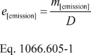Where: e[emission] = emission rate over the test interval. m[emission] = emission mass over the test interval. D = the measured driving distance over the test interval. Example: mNOx = 0.3177 g DHFET = 10.19 miles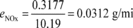(e) Calculate the emission mass of each gaseous pollutant using the following equation: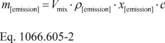Where: m[emission] = emission mass over the test interval. Vmix = total dilute exhaust volume over the test interval, corrected to standard reference conditions, and corrected for any volume removed for emission sampling and for any volume change from adding secondary dilution air. p[emission] = density of the appropriate chemical species as given in § 1066.1005(f). x[emission] = measured emission concentration in the sample, after dry-to-wet and background corrections. c = 10−2 for emission concentrations in %, and 10−6 for emission concentrations in ppm. Example: Vmix = 170.878 m 3 (from paragraph (f) of this section) rNOx = 1913 g/m 3 xNOx = 0.9721 ppm c = 10−6 mNOx = 170.878·1913·0.9721·10−6 = 0.3177 g

(f) Calculation of the emission mass of PM, mPM, is dependent on how many PM filters you use, as follows:

(1) Except as otherwise specified in this paragraph (f), calculate mPM using the following equation: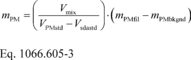Where: mPM = mass of particulate matter emissions over the test interval, as described in § 1066.815(b)(1), (2), and (3). Vmix = total dilute exhaust volume over the test interval, corrected to standard reference conditions, and corrected for any volume removed for emission sampling and for any volume change from adding secondary dilution air. For partial-flow dilution systems, set Vmix equal to the total exhaust volume over the test interval, corrected to standard reference conditions. VPMstd = total volume of dilute exhaust sampled through the filter over the test interval, corrected to standard reference conditions. Vsdastd = total volume of secondary dilution air sampled through the filter over the test interval, corrected to standard reference conditions. For partial-flow dilution systems, set Vsdastd equal to total dilution air volume over the test interval, corrected to standard reference conditions. mPMfil = mass of particulate matter emissions on the filter over the test interval. mPMbkgnd = mass of particulate matter on the background filter. Example: Vmix = 170.878 m 3 (from paragraph (g) of this section) VPMstd = 0.925 m 3 (from paragraph (g) of this section) Vsdastd = 0.527 m 3 (from paragraph (g) of this section) mPMfil = 0.0000045 g mPMbkgnd = 0.0000014 g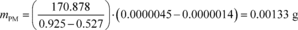(2) If you sample PM onto a single filter as described in § 1066.815(b)(4)(i) or (b)(4)(ii) (for constant volume samplers), calculate mPM using the following equation: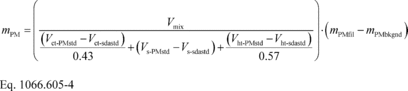Where: mPM = mass of particulate matter emissions over the entire FTP. Vmix = total dilute exhaust volume over the test interval, corrected to standard reference conditions, and corrected for any volume removed for emission sampling and for any volume change from adding secondary dilution air. V[interval]-PMstd = total volume of dilute exhaust sampled through the filter over the test interval (ct = cold transient, s = stabilized, ht = hot transient), corrected to standard reference conditions. V[interval]-sdastd = total volume of secondary dilution air sampled through the filter over the test interval (ct = cold transient, s = stabilized, ht = hot transient), corrected to standard reference conditions. mPMfil = mass of particulate matter emissions on the filter over the test interval. mPMbkgnd = mass of particulate matter on the background filter over the test interval. Example: Vmix = 633.691 m 3 Vct-PMstd = 0.925 m 3 Vct-sdastd = 0.527 m 3 Vs-PMstd = 1.967 m 3 Vs-sdastd = 1.121 m 3 Vht-PMstd = 1.122 m 3 Vht-sdastd = 0.639 m 3 mPMfil = 0.0000106 g mPMbkgnd = 0.0000014 g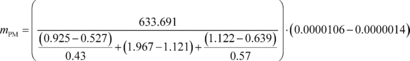mPM = 0.00222 g

(3) If you sample PM onto a single filter as described in § 1066.815(b)(4)(ii) (for partial flow dilution systems), calculate mPM using the following equation: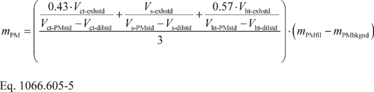Where: mPM = mass of particulate matter emissions over the entire FTP. V[interval]-exhstd = total engine exhaust volume over the test interval (ct = cold transient, s = stabilized, ht = hot transient), corrected to standard reference conditions, and corrected for any volume removed for emission sampling. V[interval]-PMstd = total volume of dilute exhaust sampled through the filter over the test interval (ct = cold transient, s = stabilized, ht = hot transient), corrected to standard reference conditions. V[interval]-dilstd = total volume of dilution air over the test interval (ct = cold transient, s = stabilized, ht = hot transient), corrected to standard reference conditions and for any volume removed for emission sampling. mPMfil = mass of particulate matter emissions on the filter over the test interval. mPMbkgnd = mass of particulate matter on the background filter over the test interval. Example: Vct-exhstd = 5.55 m 3 Vct-PMstd = 0.526 m 3 Vct-dilstd = 0.481 m 3 Vs-exhstd = 9.53 m 3 Vs-PMstd = 0.903 m 3 Vs-dilstd = 0.857 m 3 Vht-exhstd = 5.54 m 3 Vht-PMstd = 0.527 m 3 Vht-dilstd = 0.489 m 3 mPMfil = 0.0000106 g mPMbkgnd = 0.0000014 g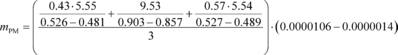mPM = 0.00269 g

(4) If you sample PM onto a single filter as described in § 1066.815(b)(5)(i) or (b)(5)(ii) (for constant volume samplers), calculate mPM using the following equation: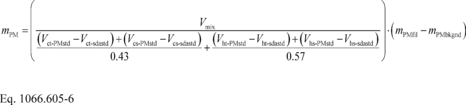Where: mPM = mass of particulate matter emissions over the entire FTP. Vmix = total dilute exhaust volume over the test interval, corrected to standard reference conditions, and corrected for any volume removed for emission sampling and for any volume change from secondary dilution air. V[interval]-PMstd = total volume of dilute exhaust sampled through the filter over the test interval (ct = cold transient, cs = cold stabilized, ht = hot transient, hs = hot stabilized), corrected to standard reference conditions. V[interval]-sdastd = total volume of secondary dilution air sampled through the filter over the test interval (ct = cold transient, cs = cold stabilized, ht = hot transient, hs = hot stabilized), corrected to standard reference conditions. mPMfil = mass of particulate matter emissions on the filter over the test interval. mPMbkgnd = mass of particulate matter on the background filter over the test interval. Example: Vmix = 972.121 m 3 Vct-PMstd = 0.925 m 3 Vct-sdastd = 0.529 m 3 Vcs-PMstd = 1.968 m 3 Vcs-sdastd = 1.123 m 3 Vht-PMstd = 1.122 m 3 Vht-sdastd = 0.641 m 3 Vhs-PMstd = 1.967 m 3 Vhs-sdastd = 1.121 m 3 mPMfil = 0.0000229 g mPMbkgnd = 0.0000014 g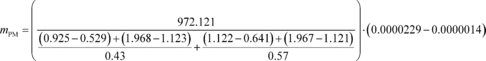mPM = 0.00401 g

(5) If you sample PM onto a single filter as described in § 1066.815(b)(5)(ii) (for partial flow dilution systems), calculate mPM using the following equation: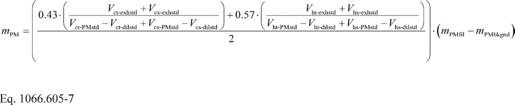Where: mPM = mass of particulate matter emissions over the entire FTP. V[interval]-exhstd = total engine exhaust volume over the test interval (ct = cold transient, cs = cold stabilized, ht = hot transient, hs = hot stabilized), corrected to standard reference conditions, and corrected for any volume removed for emission sampling. V[interval]-PMstd = total volume of dilute exhaust sampled through the filter over the test interval (ct = cold transient, cs = cold stabilized, ht = hot transient, hs = hot stabilized), corrected to standard reference conditions. V[interval]-dilstd = total volume of dilution air over the test interval (ct = cold transient, cs = cold stabilized, ht = hot transient, hs = hot stabilized), corrected to standard reference conditions and for any volume removed for emission sampling. mPMfil = mass of particulate matter emissions on the filter over the test interval. mPMbkgnd = mass of particulate matter on the background filter over the test interval. Example: Vct-exhstd = 5.55 m 3 Vct-PMstd = 0.526 m 3 Vct-dilstd = 0.481 m 3 Vcs-exhstd = 9.53 m 3 Vcs-PMstd = 0.903 m 3 Vcs-dilstd = 0.857 m 3 Vht-exhstd = 5.54 m 3 Vht-PMstd = 0.527 m 3 Vht-dilstd = 0.489 m 3 Vhs-exhstd = 9.54 m 3 Vhs-PMstd = 0.902 m 3 Vhs-dilstd = 0.856 m 3 mPMfil = 0.0000229 g mPMbkgnd = 0.0000014 g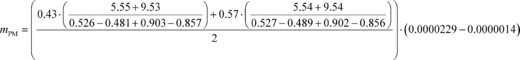mPM = 0.00266 g

(g) This paragraph (g) describes how to correct flow and flow rates to standard reference conditions and provides an example for determining Vmix based on CVS total flow and the removal of sample flow from the dilute exhaust gas. You may use predetermined nominal values for removed sample volumes, except for flows used for batch sampling.

(1) Correct flow and flow rates to standard reference conditions as needed using the following equation: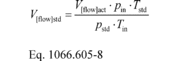Where: V[flow]std = total flow volume at the flow meter, corrected to standard reference conditions. V[flow]act = total flow volume at the flow meter at test conditions. pin = absolute static pressure at the flow meter inlet, measured directly or calculated as the sum of atmospheric pressure plus a differential pressure referenced to atmospheric pressure. Tstd = standard temperature. pstd = standard pressure. Tin = temperature of the dilute exhaust sample at the flow meter inlet. Example: VPMact = 1.071 m 3 pin = 101.7 kPa Tstd = 293.15 K pstd = 101.325 kPa Tin = 340.5 K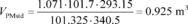(2) The following example provides a determination of Vmix based on CVS total flow and the removal of sample flow from one dilute exhaust gas analyzer and one PM sampling system that is utilizing secondary dilution. Note that your Vmix determination may vary from Eq. 1066.605-7 based on the number of flows that are removed from your dilute exhaust gas and whether your PM sampling system is using secondary dilution. For this example, Vmix is governed by the following equation: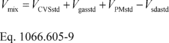Where: VCVSstd = total dilute exhaust volume over the test interval at the flow meter, corrected to standard reference conditions. Vgasstd = total volume of sample flow through the gaseous emission bench over the test interval, corrected to standard reference conditions. VPMstd = total volume of dilute exhaust sampled through the filter over the test interval, corrected to standard reference conditions. Vsdastd = total volume of secondary dilution air flow sampled through the filter over the test interval, corrected to standard reference conditions. Example:

Using Eq. 1066.605-8:

VCVSstd = 170.451 m 3, where VCVSact = 170.721 m 3, pin = 101.7 kPa, and Tin = 294.7 K Using Eq. 1066.605-8: Vgasstd = 0.028 m 3, where Vgasact = 0.033 m 3, pin = 101.7 kPa, and Tin = 340.5 K Using Eq. 1066.605-8: VPMstd = 0.925 m 3, where VPMact = 1.071 m 3, pin = 101.7 kPa, and Tin = 340.5 K Using Eq. 1066.605-8: Vsdastd = 0.527 m 3, where Vsdaact = 0.531 m 3, pin = 101.7 kPa, and Tin = 296.3 K Vmix = 170.451 + 0.028 + 0.925 − 0.527 = 170.878 m 3

(h) Calculate total flow volume over a test interval, V[flow], for a CVS or exhaust gas sampler as follows:

(1) Varying versus constant flow rates. The calculation methods depend on differentiating varying and constant flow, as follows:

(i) We consider the following to be examples of varying flows that require a continuous multiplication of concentration times flow rate: raw exhaust, exhaust diluted with a constant flow rate of dilution air, and CVS dilution with a CVS flow meter that does not have an upstream heat exchanger or electronic flow control.

(ii) We consider the following to be examples of constant exhaust flows: CVS diluted exhaust with a CVS flow meter that has an upstream heat exchanger, an electronic flow control, or both.

(2) Continuous sampling. For continuous sampling, you must frequently record a continuously updated flow signal. This recording requirement applies for both varying and constant flow rates.

(i) Varying flow rate. If you continuously sample from a varying exhaust flow rate, calculate V[flow] using the following equation: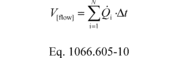Where: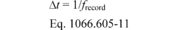Example: N = 505 Q CVS1 = 0.276 m 3/s Q CVS2 = 0.294 m 3/s frecord = 1 Hz Using Eq. 1066.605-11, Δt = 1/1 = 1 s VCVS = (0.276 + 0.294 + ... + Q CVS505)·1 VCVS = 170.721 m 3

(ii) Constant flow rate. If you continuously sample from a constant exhaust flow rate, use the same calculation described in paragraph (h)(2)(i) of this section or calculate the mean flow recorded over the test interval and treat the mean as a batch sample, as described in paragraph (h)(3)(ii) of this section.

(3) Batch sampling. For batch sampling, calculate total flow by integrating a varying flow rate or by determining the mean of a constant flow rate, as follows:

(i) Varying flow rate. If you proportionally collect a batch sample from a varying exhaust flow rate, integrate the flow rate over the test interval to determine the total flow from which you extracted the proportional sample, as described in paragraph (h)(2)(i) of this section.

(ii) Constant flow rate. If you batch sample from a constant exhaust flow rate, extract a sample at a proportional or constant flow rate and calculate V[flow] from the flow from which you extract the sample by multiplying the mean flow rate by the time of the test interval using the following equation: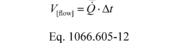Example: Q CVS = 0.338 m 3/s Δt = 505 s VCVS = 0.338·505 VCVS = 170.69 m 3 [79 FR 23823, Apr. 28, 2014, as amended at 80 FR 9121, Feb. 19, 2015; 81 FR 74203, Oct. 25, 2016]